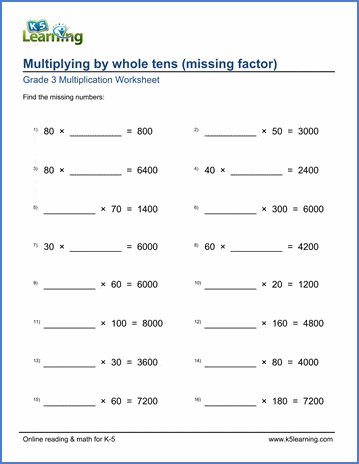# Multiplying by whole tens (missing factors)

## Math worksheets: Multiplying by whole tens (1-100) with missing factors

Below are six versions of our grade 3 multiplication worksheet on multiplying whole tens by whole tens (or single digits); students must determine the missing factor in each equation. These worksheets are pdf files.## More multiplication worksheets

Find all of our multiplication worksheets, from basic multiplication facts to multiplying multi-digit whole numbers in columns.

## What is K5?

K5 Learning offers reading and math worksheets, workbooks and an online reading and math program for kids in kindergarten to grade 5.  We help your children build good study habits and excel in school.